Static Electricity - Lesson 4 - Electric Fields

# Electric Field IntensityIn the previous section of Lesson 4, the concept of an electric field was introduced. It was stated that the electric field concept arose in an effort to explain action-at-a-distance forces. All charged objects create an electric field that extends outward into the space that surrounds it. The charge alters that space, causing any other charged object that enters the space to be affected by this field. The strength of the electric field is dependent upon how charged the object creating the field is and upon the distance of separation from the charged object. In this section of Lesson 4, we will investigate electric field from a numerical viewpoint - the electric field strength.

### The Force per Charge Ratio

Electric field strength is a vector quantity; it has both magnitude and direction. The magnitude of the electric field strength is defined in terms of how it is measured. Let's suppose that an electric chargecan be denoted by the symbol Q. This electric charge creates an electric field; since Q is the source of the electric field, we will refer to it as the source charge. The strength of the source charge's electric field could be measured by any other charge placed somewhere in its surroundings. The charge that is used to measure the electric field strength is referred to as a test charge since it is used to test the field strength. The test charge has a quantity of charge denoted by the symbol q. When placed within the electric field, the test charge will experience an electric force - either attractive or repulsive. As is usually the case, this force will be denoted by the symbol F. The magnitude of the electric field is simply defined as the force per charge on the test charge.If the electric field strength is denoted by the symbol E, then the equation can be rewritten in symbolic form as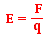.

The standard metric units on electric field strength arise from its definition. Since electric field is defined as a force per charge, its units would be force units divided by charge units. In this case, the standard metric units are Newton/Coulomb or N/C.

In the above discussion, you will note that two charges are mentioned - the source charge and the test charge. Two charges would always be necessary to encounter a force. In the electric world, it takes two to attract or repel. The equation for electric field strength (E) has one of the two charge quantities listed in it. Since there are two charges involved, a student will have to be ultimately careful to use the correct charge quantity when computing the electric field strength. The symbol q in the equation is the quantity of charge on the test charge (not the source charge). Recall that the electric field strength is defined in terms of how it is measured or tested; thus, the test charge finds its way into the equation. Electric field is the force per quantity of charge on the test charge.

The electric field strength is not dependent upon the quantity of charge on the test charge. If you think about that statement for a little while, you might be bothered by it. (Of course if you don't think at all - ever - nothing really bothers you. Ignorance is bliss.) After all, the quantity of charge on the test charge (q) is in the equation for electric field. So how could electric field strength not be dependent upon q if q is in the equation? Good question. But if you think about it a little while longer, you will be able to answer your own question. (Ignorance might be bliss. But with a little extra thinking you might achieve insight, a state much better than bliss.) Increasing the quantity of charge on the test charge - say, by a factor of 2 - would increase the denominator of the equation by a factor of 2. But according to Coulomb's law, more charge also means more electric force (F). In fact, a twofold increase in q would be accompanied by a twofold increase in F. So as the denominator in the equation increases by a factor of two (or three or four), the numerator increases by the same factor. These two changes offset each other such that one can safely say that the electric field strength is not dependent upon the quantity of charge on the test charge. So regardless of what test charge is used, the electric field strength at any given location around the source charge Q will be measured to be the same.

### Another Electric Field Strength Formula

The above discussion pertained to defining electric field strength in terms of how it is measured. Now we will investigate a new equation that defines electric field strength in terms of the variables that affect the electric field strength. To do so, we will have to revisit the Coulomb's law equation. Coulomb's law states that the electric force between two charges is directly proportional to the product of their charges and inversely proportional to the square of the distance between their centers. When applied to our two charges - the source charge (Q) and the test charge (q) - the formula for electric force can be written as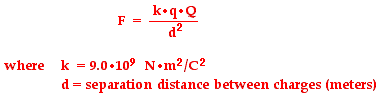If the expression for electric force as given by Coulomb's law is substituted for force in the above E =F/q equation, a new equation can be derived as shown below.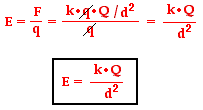Note that the derivation above shows that the test charge q was canceled from both numerator and denominator of the equation. The new formula for electric field strength (shown inside the box) expresses the field strength in terms of the two variables that affect it. The electric field strength is dependent upon the quantity of charge on the source charge (Q) and the distance of separation (d) from the source charge.

### An Inverse Square Law

Like all formulas in physics, the formulas for electric field strength can be used to algebraically solve physics word problems. And like all formulas, these electric field strength formulas can also be used to guide our thinking about how an alteration of one variable might (or might not) affect another variable. One feature of this electric field strength formula is that it illustrates an inverse square relationship between electric field strength and distance. The strength of an electric field as created by source charge Q is inversely related to square of the distance from the source. This is known as an inverse square law.

Electric field strength is location dependent, and its magnitude decreases as the distance from a location to the source increases. And by whatever factor the distance is changed, the electric field strength will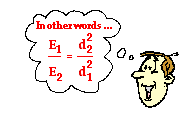change inversely by the square of that factor. So if separation distance increases by a factor of 2, the electric field strength decreases by a factor of 4 (2^2). If the separation distance increases by a factor of 3, the electric field strength decreases by a factor of 9 (3^2). If the separation distance increases by a factor of 4, the electric field strength decreases by a factor of 16 (4^2). And finally, if separation distance decreases by a factor of 2, the electric field strength increases by a factor of 4 (2^2).

Use this principle of the inverse square relationship between electric field strength and distance to answer the first three questions in the Check Your Understanding section below.

### The Stinky Field Analogy Revisited

In the previous section of Lesson 4, a somewhat crude yet instructive analogy was presented - the stinky field analogy. The analogy compares the concept of an electric field surrounding a source charge to the stinky field that surrounds an infant's stinky diaper. Just as every stinky diaper creates a stinky field, every electric charge creates an electric field. And if you want to know the strength of the stinky field, you simply use a stinky detector - a nose that (as far as I have experienced) always responds in a repulsive manner to the stinky source. In the same way, if you want to know the strength of an electric field, you simply use a charge detector - a test charge that will respond in an attractive or repulsive manner to the source charge. And of course the strength of the field is proportional to the effect upon the detector. A more sensitive detector (a better nose or a more charged test charge) will sense the effect more intensely. Yet the field strength is defined as the effect (or force) per sensitivity of the detector; so the field strength of a stinky diaper or of an electric charge is not dependent upon the sensitivity of the detector.

If you measure the diaper's stinky field, it only makes sense that it would not be affected by how stinky you are. A person measuring the strength of a diaper's stinky field can create their own field, the strength of which is dependent upon how stinky they are. But that person's field is not to be confused with the diaper's stinky field. The diaper's stinky field depends on how stinky the diaper is. In the same way, the strength of a source charge's electric field is dependent upon how charged up the source charge is. Furthermore, just as with the stinky field, our electric field equation shows that as you get closer and closer to the source of the field, the effect becomes greater and greater and the electric field strength increases.

The stinky field analogy proves useful in conveying both the concept of an electric field and the mathematics of an electric field. Conceptually, it illustrates how the source of a field can affect the surrounding space and exert influences upon sensitive detectors in that space. And mathematically, it illustrates how the strength of the field is dependent upon the source and the distance from the source and independent of any characteristic having to do with the detector.

### The Direction of the Electric Field Vector

As mentioned earlier, electric field strength is a vector quantity. Unlike a scalar quantity, a vector quantity is not fully described unless there is a direction associated with it. The magnitude of the electric field vector is calculated as the force per charge on any given test charge located within the electric field. The force on the test charge could be directed either towards the source charge or directly away from it. The precise direction of the force is dependent upon whether the test charge and the source charge have the same type of charge (in which repulsion occurs) or the opposite type of charge (in which attraction occurs). To resolve the dilemma of whether the electric field vector is directed towards or away from the source charge, a convention has been established. The worldwide convention that is used by scientists is to define the direction of the electric field vector as the direction that a positive test charge is pushed or pulled when in the presence of the electric field. By using the convention of a positive test charge, everyone can agree upon the direction of E.

Given this convention of a positive test charge, several generalities can be made about the direction of the electric field vector. A positive source charge would create an electric field that would exert a repulsive effect upon a positive test charge. Thus, the electric field vector would always be directed away from positively charged objects. On the other hand, a positive test charge would be attracted to a negative source charge. Therefore, electric field vectors are always directed towards negatively charged objects. You might test your understanding of electric field directions by attempting questions 6 and 7 below.

### We Would Like to Suggest ...Sometimes it isn't enough to just read about it. You have to interact with it! And that's exactly what you do when you use one of The Physics Classroom's Interactives. We would like to suggest that you combine the reading of this page with the use of our Put the Charge in the Goal Interactive and/or our Electrostatics Landscapes Interactive. Both Interactives can be found in the Physics Interactives section of our website. Both Interactives provide engaging environments for exploring electric fields and action-at-a-distance.

Use your understanding to answer the following questions. When finished, click the button to view the answers.

1. Charge Q acts as a point charge to create an electric field. Its strength, measured a distance of 30 cm away, is 40 N/C. What is the magnitude of the electric field strength that you would expect to be measured at a distance of ...

a. 60 cm away?

b. 15 cm away?

c. 90 cm away?

d. 3 cm away?

c. 45 cm away?

2. Charge Q acts as a point charge to create an electric field. Its strength, measured a distance of 30 cm away, is 40 N/C. What would be the electric field strength ...

a. 30 cm away from a source with charge 2Q?

b. 30 cm away from a source with charge 3Q?

c. 60 cm away from a source with charge 2Q?

d. 15 cm away from a source with charge 2Q?

e. 150 cm away from a source with charge 0.5Q?

3. Use your understanding of electric field strength to complete the following table.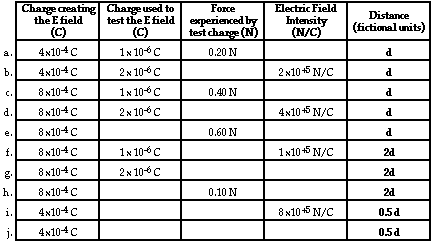4. In the table above, identify at least two rows that illustrate that the strength of the electric field vector is ...

a. directly related to the quantity of charge on the source charge (Q).

b. inversely related to the square of the separation distance (d).

c. independent of the quantity of charge on the test charge (q).

5. The following unit is certainly not the standard unit for expressing the quantity electric field strength.

kg • m / s2 / C

However, it could be an acceptable unit for E. Use unit analysis to identify whether the above set of units is an acceptable unit for electric field strength.

6. It is observed that Balloon A is charged negatively. Balloon B exerts a repulsive effect upon balloon A. Would the electric field vector created by balloon B be directed towards B or away from B? ___________ Explain your reasoning.

7. A negative source charge (Q) is shown in the diagram below. This source charge can create an electric field. Various locations within the field are labeled. For each location, draw an electric field vector in the appropriate direction with the appropriate relative magnitude. That is, draw the length of the E vector long wherever the magnitude is large and short wherever the magnitude is small.Next Section: